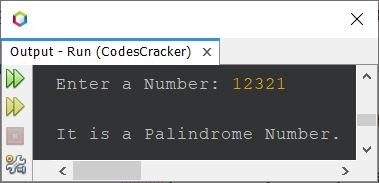# Java Program to Check Palindrome or Not

• Java Program to Check Palindrome Number or Not
• Java Program to Check Palindrome String or Not

Note - A number whose reverse is equal to the number itself, is called a palindrome number. For example, 12321, 121, 1001 etc. are palindrome numbers.

Note - A string whose reverse is equal to the original string, is called as a palindrome string. For example, radar, madam, refer, level, reviver etc. are all palindrome strings.

## Check Palindrome Number in Java

The question is, write a Java program to check whether a given number is a palindrome number or not. The number must be received by user at run-time of the program. The program given below is its answer:

```import java.util.Scanner;

public class CodesCracker
{
public static void main(String[] args)
{
int num, rev=0, rem, temp;
Scanner scan = new Scanner(System.in);

System.out.print("Enter a Number: ");
num = scan.nextInt();

temp = num;
while(temp!=0)
{
rem = temp%10;
rev = (rev*10) + rem;
temp = temp/10;
}

if(num==rev)
System.out.println("\nIt is a Palindrome Number.");
else
System.out.println("\nIt is not a Palindrome Number.");
}
}```

The snapshot given below shows the sample run of above Java program on checking whether a given number is a palindrome number or not, with user input 12321:The above program can also be created in this way:

```import java.util.Scanner;

public class CodesCracker
{
public static void main(String[] args)
{
int rev=0;
Scanner scan = new Scanner(System.in);

System.out.print("Enter a Number: ");
int num = scan.nextInt();

int temp = num;
while(temp!=0)
{
rev = (rev*10) + (temp%10);
temp /= 10;
}

if(num==rev)
System.out.println("\n" +num+ " is a Palindrome Number.");
else
System.out.println("\n" +num+ " is not a Palindrome Number.");
}
}```

Here is its sample run with user input 1245## Check Palindrome String in Java

The question is, write a Java program to check whether a string entered by user at run-time, is a palindrome string or not. The program given below is its answer:

```import java.util.Scanner;

public class CodesCracker
{
public static void main(String[] args)
{
String str, strReverse="";
char ch;
int i, strLen;
Scanner scan = new Scanner(System.in);

System.out.print("Enter the String: ");
str = scan.nextLine();

strLen = str.length();
i = (strLen-1);
while(i>=0)
{
ch = str.charAt(i);
strReverse = strReverse + ch;
i--;
}

if(str.equals(strReverse))
System.out.println("\n\"" +str+ "\" is a Palindrome String.");
else
System.out.println("\n\"" +str+ "\" is not a Palindrome String.");
}
}```

The sample run of above program with user input radar is shown in the snapshot given below:#### Same Program in Other Languages

Java Online Test

« Previous Program Next Program »